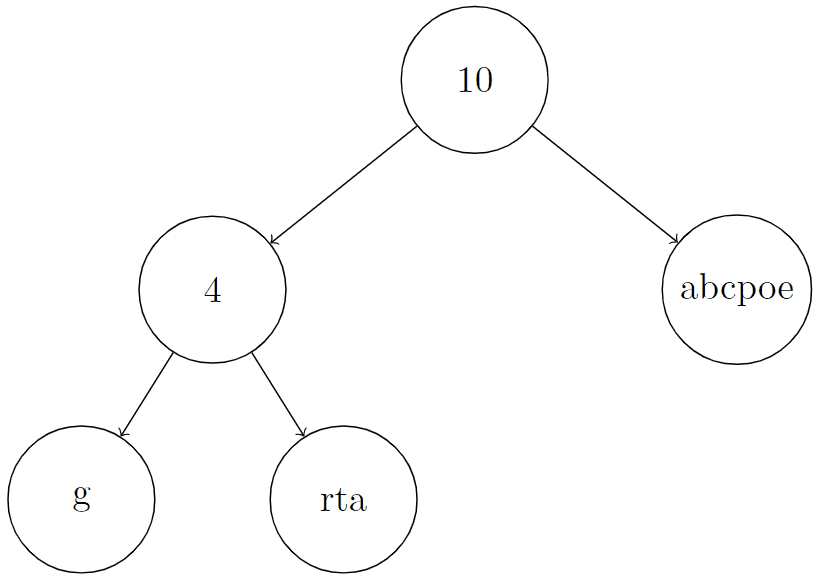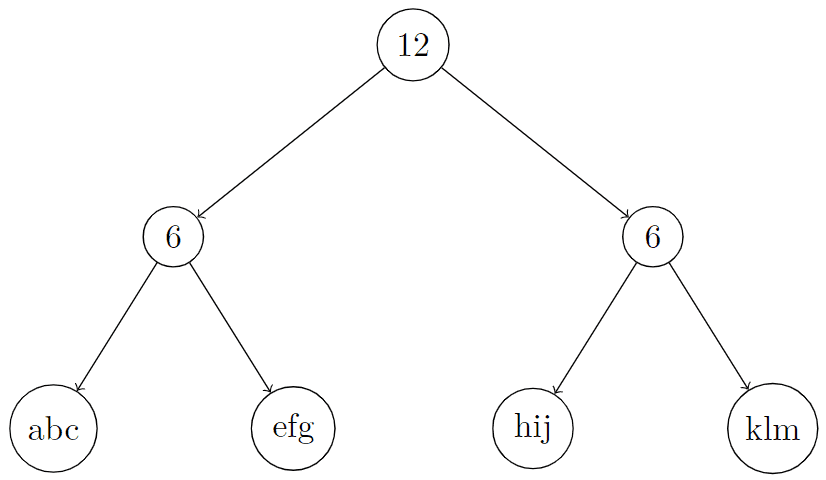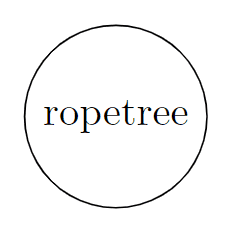# 2689. Extract Kth Character From The Rope Tree

## Description

You are given the root of a binary tree and an integer k. Besides the left and right children, every node of this tree has two other properties, a string node.val containing only lowercase English letters (possibly empty) and a non-negative integer node.len. There are two types of nodes in this tree:

• Leaf: These nodes have no children, node.len = 0, and node.val is some non-empty string.
• Internal: These nodes have at least one child (also at most two children), node.len > 0, and node.val is an empty string.

The tree described above is called a Rope binary tree. Now we define S[node] recursively as follows:

• If node is some leaf node, S[node] = node.val,
• Otherwise if node is some internal node, S[node] = concat(S[node.left], S[node.right]).

Return k-th character of the string S[root].

Note: If s and p are two strings, concat(s, p) is a string obtained by concatenating p to s. For example, concat("ab", "zz") = "abzz".

Example 1:

Input: root = [10,4,"abcpoe","g","rta"], k = 6
Output: "b"
Explanation: In the picture below, we put an integer on internal nodes that represents node.len, and a string on leaf nodes that represents node.val.
You can see that S[root] = concat(concat("g", "rta"), "abcpoe") = "grtaabcpoe". So S[root], which represents 6th character of it, is equal to "b".Example 2:

Input: root = [12,6,6,"abc","efg","hij","klm"], k = 3
Output: "c"
Explanation: In the picture below, we put an integer on internal nodes that represents node.len, and a string on leaf nodes that represents node.val.
You can see that S[root] = concat(concat("abc", "efg"), concat("hij", "klm")) = "abcefghijklm". So S[root], which represents the 3rd character of it, is equal to "c".Example 3:

Input: root = ["ropetree"], k = 8
Output: "e"
Explanation: In the picture below, we put an integer on internal nodes that represents node.len, and a string on leaf nodes that represents node.val.
You can see that S[root] = "ropetree". So S[root], which represents 8th character of it, is equal to "e".Constraints:

• The number of nodes in the tree is in the range [1, 103]
• node.val contains only lowercase English letters
• 0 <= node.val.length <= 50
• 0 <= node.len <= 104
• for leaf nodes, node.len = 0 and node.val is non-empty
• for internal nodes, node.len > 0 and node.val is empty
• 1 <= k <= S[root].length

## Solutions

• /**
* Definition for a rope tree node.
* class RopeTreeNode {
*     int len;
*     String val;
*     RopeTreeNode left;
*     RopeTreeNode right;
*     RopeTreeNode() {}
*     RopeTreeNode(String val) {
*         this.len = 0;
*         this.val = val;
*     }
*     RopeTreeNode(int len) {
*         this.len = len;
*         this.val = "";
*     }
*     RopeTreeNode(int len, TreeNode left, TreeNode right) {
*         this.len = len;
*         this.val = "";
*         this.left = left;
*         this.right = right;
*     }
* }
*/
class Solution {
public char getKthCharacter(RopeTreeNode root, int k) {
return dfs(root).charAt(k - 1);
}

private String dfs(RopeTreeNode root) {
if (root == null) {
return "";
}
if (root.val.length() > 0) {
return root.val;
}
String left = dfs(root.left);
String right = dfs(root.right);
return left + right;
}
}

• /**
* Definition for a rope tree node.
* struct RopeTreeNode {
*     int len;
*     string val;
*     RopeTreeNode *left;
*     RopeTreeNode *right;
*     RopeTreeNode() : len(0), val(""), left(nullptr), right(nullptr) {}
*     RopeTreeNode(string s) : len(0), val(std::move(s)), left(nullptr), right(nullptr) {}
*     RopeTreeNode(int x) : len(x), val(""), left(nullptr), right(nullptr) {}
*     RopeTreeNode(int x, RopeTreeNode *left, RopeTreeNode *right) : len(x), val(""), left(left), right(right) {}
* };
*/
class Solution {
public:
char getKthCharacter(RopeTreeNode* root, int k) {
function<string(RopeTreeNode* root)> dfs = [&](RopeTreeNode* root) -> string {
if (root == nullptr) {
return "";
}
if (root->len == 0) {
return root->val;
}
string left = dfs(root->left);
string right = dfs(root->right);
return left + right;
};
return dfs(root)[k - 1];
}
};

• # Definition for a rope tree node.
# class RopeTreeNode(object):
#     def __init__(self, len=0, val="", left=None, right=None):
#         self.len = len
#         self.val = val
#         self.left = left
#         self.right = right
class Solution:
def getKthCharacter(self, root: Optional[object], k: int) -> str:
def dfs(root):
if root is None:
return ""
if root.len == 0:
return root.val
return dfs(root.left) + dfs(root.right)

return dfs(root)[k - 1]


• /**
* Definition for a rope tree node.
* type RopeTreeNode struct {
* 	   len   int
* 	   val   string
* 	   left  *RopeTreeNode
* 	   right *RopeTreeNode
* }
*/
func getKthCharacter(root *RopeTreeNode, k int) byte {
var dfs func(root *RopeTreeNode) string
dfs = func(root *RopeTreeNode) string {
if root == nil {
return ""
}
if root.len == 0 {
return root.val
}
left, right := dfs(root.left), dfs(root.right)
return left + right
}
return dfs(root)[k-1]
}